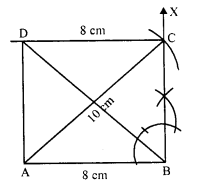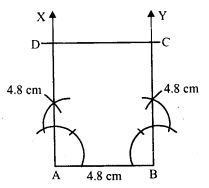# RD Sharma Class 8 Solutions Chapter 17 Understanding Shapes III Ex 17.3

In this chapter, we provide RD Sharma Class 8 Solutions Chapter 17 Understanding Shapes Ex 17.3 for English medium students, Which will very helpful for every student in their exams. Students can download the latest RD Sharma Class 8 Solutions Chapter 17 Understanding Shapes Ex 17.3 Maths pdf, free RD Sharma Class 8 Solutions Chapter 17 Understanding Shapes Ex 17.3 book pdf download. Now you will get step by step solution to each question.

### RD Sharma Solutions for Class 8 Chapter 17 Understanding Shapes Ex 17.3Download PDF

Question 1.
Which of the following statements are true for a rectangle ?
(i) It has two pairs of equal sides.
(ii) It has all its sides of equal length.
(iii) Its diagonals are equal.
(iv) Its diagonals bisect each other.
(v) Its diagonals are perpendicular.
(vi) Its diagonals are perpendicular and bisect each other.
(vii) Its diagonals are equal and bisect each other.
(viii) Its diagonals are equal and perpendicular, and bisect each other.
(ix) All rectangles are squares.
(x) All rhombuses are parallelograms.
(xi) All squares are rhombuses and also rectangles.
(xii) All squares are not parallelograms.
Solution:
(i) True.
(ii) False. (Only pair of opposite sides is equal)
(iii) True
(iv) True
(v) False (Diagonals are not perpendicular)
(vi) False (Diagonals are not perpendicular to each other)
(vii) True
(viii) False (Diagonals are equal but not perpendicular)
(ix) False (All rectangles are not square but a special type can be a square)
(x) True
(xi) True
(xii) False (All squares are parallelograms because their opposite sides are parallel and equal)

Question 2.
Which of the following statements are true for a square ?
(i) It is a rectangle.
(ii) It has all its sides of equal length.
(iii) Its diagonals bisect each other at right angle.
(iv) Its diagonals are equal to its sides.
Solution:
(i) True
(ii) True
(iii) True
(iv) False (Each diagonal of a square is greater than its side)

Question 3.
Fill in the blanks in each of the following so as to make the statement true :
(i) A rectangle is a parallelogram in which ……..
(ii) A square is a rhombus in which ……….
(iii) A square is a rectangle in which ………
Solution:
(i) A rectangle is a parallelogram in which one angle is right angle.
(ii) A square is a rhombus in which one angle is right angle.
(iii) A square is a rectangle in which adjacent sides are equal.

Question 4.
A window frame has one diagonal longer than the other. Is the window frame a rectangle ? Why or why not ?
Solution:
No, it is not a rectangle as rectangle has diagonals of equal length.

Question 5.
In a rectangle ABCD, prove that ∆ACB = ∆CAD.
Solution:
In rectangle ABCD, AC is its diagonal.AB = CD (Opposite sides of a rectangle)
AC = AC (Common)

Question 6.
The sides of a rectangle are in the ratio 2 : 3 and its perimeter is 20 cm. Draw the rectangle.
Solution:
Perimeter of a rectangle = 20 cm
Ratio in the sides = 2 : 3Then length (b) = 3x
Perimeter = 2 (l + b)
⇒ 20 = 2 (2x + 3x)
⇒ 4x + 6x = 20
⇒ 10x = 20
⇒ x = 2010 = 2
Length = 3x = 3 x 2 = 6
and breadth = 2x = 2 x 2 = 4 cm
Steps of construction:
(i) Draw a line segment AB = 6 cm.
(ii) At A and B draw perpendicular AX and BY.
(iii) Cut off from AX and BY,
AD = BC = 4 cm.
(iv) Join CD.
Then ABCD is the required rectangle.

Question 7.
The sides of a rectangle are the ratio 4 : 5. Find its sides if the perimeter is 90 cm.
Solution:
Perimeter of a rectangle = 90 cm.Ratio in sides = 4 : 5
Let first side = 4x
Then second side = 5x
Perimeter = 2 (l + b)
⇒ 2 (4x + 5x) = 90
⇒ 2 x 9x = 90
⇒ 18x = 90
⇒ x = 5
First side = 4x = 4 x 5 = 20 cm
and second side = 5x = 5 x 5 = 25 cm

Question 8.
Find the length of the diagonal of a rectangle whose sides are 12 cm and 5 cm.
Solution:
In rectangle ABCD, AB = 12 cm and AD = 5 cmBD is its diagonal.
Now, in right angled ∆ABD,
BD² = AB² + AD² (Pythagoras theorem)
= (12)² + (5)² = 144 + 25 = 169 = (13)²
BD = 13 cm
Length of diagonal = 13 cm

Question 9.
Draw a rectangle whose one side measures 8 cm and the length of each of whose diagonals is 10 cm.
Solution:
Steps of construction :
(i) Draw a line segment AB = 8 cm(ii) At B, draw a perpendicular BX
(iii) With centre A and radius 10 cm, draw an arc which intersects BX at C.
(iv) With centre C and radius equal to AB and with centre A and radius equal to BC, draw arcs which intersect at D.
(v) Join AD, AC, CD and BD.
Then ABCD is the required rectangle.

Question 10.
Draw a square whose each side measures 4.8 cm.
Solution:
Steps of construction :
(i) Draw a line segment AB = 4.8 cm.(ii) At A and B, draw perpendiculars AX and BY.
(iii) Cut off AD = BC = 4.8 cm
(iv) Join CD.
Then ABCD is the required square.

Question 11.
Identify all the quadrilaterals that have:
(i) Four sides of equal length.
(ii) Four right angles.
Solution:
(i) A quadrilateral whose four sides are equal can be a square or a rhombus.
(ii) A quadrilateral whose four angle are right angle each can be a square or a rectangle.

Question 12.
Explain how a square is
(ii) a parallelogram
(iii) a rhombus
(iv) a rectangle ?
Solution:
(i) A square is a quadrilateral as it has four sides and four angles.
(ii) A square is a parallelogram, because its opposite sides are parallel and equal.
(iii) A square is a rhombus because it has all sides equal and opposite sides are parallel.
(iv) A square is a rectangle as its opposite sides are equal and each angle is of 90°.

Question 13.
(i) bisect each other
(ii) are perpendicular bisector of each other
(iii) are equal.
Solution:
(i) A quadrilateral whose diagonals bisect each other can be a square, rectangle, rhombus or a parallelogram.
(ii) A quadrilateral whose diagonals are perpendicular bisector of each other can be a square or a rhombus.
(iii) A quadrilateral whose diagonals are equal can be a square or a rectangle.

Question 14.
ABC is a right-angled triangle and O is the mid-point of the side opposite to the right angle. Explain why O is equidistant from A, B and C.
Solution:
In ∆ABC, ∠B = 90°.
O is the mid-point of AC i.e. OA = OC.BO is joined.
Now, we have to prove that OA = OB = OC
Produce BO to D such that OD = OB.
Join DC and DA.
In ∆AOB and ∆COD
OA = OC (O is the mid point of AC)
OB = OD (Construction)
∠AOB = ∠COD (Vertically opposite angles)
∆AOB = ∆COD (SAS condition)
AB = CD (c.p.c.t.) …..(i)
Similarly, we can prove that
∆BOC = ∆AOD
From (i) and (ii)
ABCD is a rectangle.
But diagonals of a rectangle bisect each other and are equal in length.
AC and BD bisect each other at O.
OA = OC = OB.
O is equidistant from A, B and C.

Question 15.
A mason has made a concrete slap. He needs it to be rectangular. In what different ways can he make sure that it is a rectangular ?
Solution:
By definition, a rectangle has each angle of 90° and their diagonals are equal.
The mason will check the slab whether it is a rectangular in shape by measuring that
(i) its each angle is 90°
(ii) its both diagonals are equal.

All Chapter RD Sharma Solutions For Class 8 Maths

—————————————————————————–

All Subject NCERT Exemplar Problems Solutions For Class 8

All Subject NCERT Solutions For Class 8

*************************************************

I think you got complete solutions for this chapter. If You have any queries regarding this chapter, please comment on the below section our subject teacher will answer you. We tried our best to give complete solutions so you got good marks in your exam.

If these solutions have helped you, you can also share rdsharmasolutions.in to your friends.